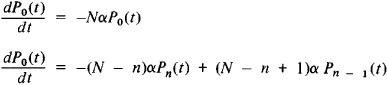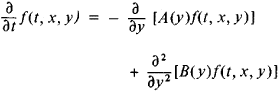# Markov process

Also found in: Dictionary, Thesaurus, Medical, Acronyms, Wikipedia.

## Markov process

[′mär‚kȯf prä·səs]
(mathematics)
A stochastic process which assumes that in a series of random events the probability of an occurrence of each event depends only on the immediately preceding outcome.
McGraw-Hill Dictionary of Scientific & Technical Terms, 6E, Copyright © 2003 by The McGraw-Hill Companies, Inc.
The following article is from The Great Soviet Encyclopedia (1979). It might be outdated or ideologically biased.

## Markov Process

an important special type of random processes, which are of great importance in applications of probability theory to various branches of natural science and technology.

An example of a Markov process is the decay of a radioactive substance. It is known that the probability of disintegration of a given atom over a short time interval dt is given by adt, where a is a constant that characterizes the rate of disintegration of the radioactive substance. This probability is independent of the fate of all the other atoms and of the age of the particular atom. Let N denote the number of atoms of a radioactive substance at some initial moment of time l = 0, and let Pn(t) denote the probability that n atoms will have disintegrated by time t. The probabilities Pn(t) satisfy the system of differential equationswhere n = 1, 2, …, N. Solving this system of equations with the initial data

P0(0) = 1 Pn(0) = 0 1 ≤ nN

we obtain

Pn(t) = CNn(1 — e-αt)ne-(N — n)αt

In this example there exist 0, 1, 2, …, N disintegrated atoms at each moment of time; their number characterizes the state of the phenomenon under study.

This example may be put into the following more general scheme. Let ω1, ω2,…,ωn… be all possible states of a given system, either finite or infinite in number. At each moment the system may be in one of these states, and over the course of time it passes randomly from one state into another. This is called a Markov process if the state of a system ωi, at a given moment is determined only by the probability Pij that after time t the system will be in state >j, this probability is independent of the course of the process in the preceding period. The probabilities Pij (t) are called transition probabilities. Under very broad conditions the transition probabilities of a Markov process satisfy a finite or infinite system of linear homogeneous ordinary differential equations.

The theory of Markov processes is based on the studies of A. A. Markov (the elder), who in his works in 1907 set forth the foundations of the study of sequences of dependent tests and sums of random variables associated with them. This line of research is now known as the theory of Markov chains. The theory treats such systems that can pass from one state to another only at fully defined moments t1, t2, …, tk, …. Let pij denote the probability that the system at moment tk + 1 will be in state oωj if it is known that at tk it was in state >i. The investigation of Markov chains can be reduced to the study of the transition probability matrices ║Pij ║.

A number of physicists and engineers have also demonstrated in their research the importance of processes in which a given system undergoes random variations as a function of a given number of continuously varying parameters (time, coordinates, and so on). Research along these lines lacked a solid logical foundation. The Soviet mathematician A. N. Kolmogorov gave a general theory and classification of Markov processes in 1930. His studies provided a logically faultless mathematical foundation of the general theory of Markov processes, encompassing, in addition to processes of the type described above, diffusion-type processes, in which the state of a system is characterized by a continuously varying coordinate of the diffusing particle.

In this case, it is natural to replace the transition probabilities by the corresponding probability density f (t,x,y). Then f (t,x,y is the probability that a particle at the point x will have a coordinate between y and y + dy after time t. Kolmogorov proved that under certain general conditions the densities f (t,x,y satisfy the partial differential equationwhich had previously been introduced for the physically important special case of a diffusion process by the German physicists A. Fokker and M. Planck. In this equation, the coefficient A (y) is the mean rate of variation of the coordinate y and the coefficient B (y) is the rate of the random variations about the mean. This equation lies at the foundations of many investigations into the theory of Markov processes in the USSR and abroad.

### REFERENCES

Markov, A. A. Izbr. trudy: Teoriia chisel, teoriia veroiatnostei. Moscow, 1951.
Kolmogorov, A N. “Ob analiticheskikh metodakh v teorii veroiatnostei.” Uspekhi matematicheskikh nauk, 1938, fasc. 5.
Feller, W. Vvedenie v teoriiu veroiatnostei i ee prilozheniia, vols. 1-2. Moscow, 1967. (Translated from English.)
Gikhman, I. I., and A. V. Skorokhod. Vvedenie v teoriiu sluchainykh protsessov. Moscow, 1965.

B. A. SEVAST’IANOV and S. KH. SIRAZHDINOV

The Great Soviet Encyclopedia, 3rd Edition (1970-1979). © 2010 The Gale Group, Inc. All rights reserved.

## Markov process

(probability, simulation)
A process in which the sequence of events can be described by a Markov chain.
This article is provided by FOLDOC - Free Online Dictionary of Computing (foldoc.org)
References in periodicals archive ?
Since the exogenous process [u.sub.t] is mean-zero and independent of the Markov process [s.sub.t], for any [y.sub.t] given by Equations (15) and (16) we have
The value for "total" leads one to reject the null hypothesis of a simple Markov process at the .005 level of significance.
One can see that the Markov process makes the convergence of solutions worse than white noise.
We denote X(t) = ([X.sub.1](t), [X.sub.2](t)) as the Markov process that represents ([X.sub.1], [X.sub.2]) at the beginning of the time t.
At first, introduce the definition of first-order Markov process. Set a sequence [{[Y.sub.n]}.sub.n[greater than or equal to]1] of random variables.
Then we assume that the connection C = [[[c.sub.ij]].sub.nxn] of the supply networks is stochastic switching, which is dependent on a continuous time Markov process. Let [[[theta].sub.t], t [greater than or equal to] 0} be a right-continuous Markov chain on a probability space taking values in a finite set S = {1, 2, ..., s} with transition probability matrix given by
Following the context of the theory of Markov processes' cycle-circuit representation, the present work arises as an attempt to investigate proper criterions regarding the properties of transience and recurrence of the corresponding Markov chain represented uniquely by directed cycles (especially by directed circuits) and weights of a random walk with jumps (having one elastic left barrier) in a fixed ergodic environment (Kalpazidou , Derriennic ).
Let [MATHEMATICAL EXPRESSION NOT REPRODUCIBLE IN ASCII] be a right-continuous Markov process on a finite state space E = {1, ..., P, [DELTA]}.
Controlled Markov Processes and Viscosity Solutions, New York and Heidelberg: Springer-Verlag, 1992.
In making these decisions, agents face uncertainty due to random growth rates of the endowment (equal to consumption in equilibrium) and the nominal price level.(3) It is assumed that the vector of these growth rates follows a stationary Markov process. At the beginning of each period, agents learn of the state of the world and visit the asset market in which one- and two-period nominal bonds are traded.

Site: Follow: Share:
Open / Close